Gas Law Problems from ChemTeam (http://dbhs.wvusd.k12.ca.us/GasLaw/KMT-Gas-Laws.html)--Copyright © 1996 by John L. Park

• Boyles Law:

• P1V1 = P2V2. This equation of P1V1 = P2V2 is the important one for solving Boyle's Law problems.

1. Boyles Law:  P1V1 = P2V2. This equation of P1V1 = P2V2 is the important one for solving Boyle's Law problems.

• Example #1: 2.00 L of a gas is at 740.0 mmHg pressure. What is its volume at standard pressure? Answer: this problem is solved by inserting values into P1V1 = P2V2

• (740.0 mmHg) (2.00 L) =(760.0 mmHg) (x)

• Multiply the left side and divide (by 760.0 mmHg) to solve for x. Note that the units of mmHg will cancel. x is a symbol for an unknown and, technically, does not carry units. So do not write x L for x liters. Keep checking to see you are using the proper equation and you have all the right values and units. Don't put a unit on the unknown.

• X =(740 mmHg) (2.00L) / (760.0 mmHg)

2. Example #2: 5.00 L of a gas is at 1.08 atm. What pressure is obtained when the volume is 10.0 L?

• Answer: use the same technique.

• 1.08 atm) (5.00 L) =(x) (10.0 L)

• X = (1.08 atm) (5.00 L) /   (10.0 L)

3. Example #3: 2.50 L of a gas was at an unknown pressure. However, at standard pressure, its volume was determined to be 8.00 L. What was the unknown pressure?

• Answer: notice the units of the pressure were not specified, so any can be used. If this were a test question, you might want to inquire of the teacher as to a possible omission of desired units. Let's use kPa since the other two units were used above. Once again, insert into P1V1 = P2V2 for the solution.

• (x) (2.50 L) = (101.325 kPa) (8.00 L)

• (101.325 kPa) (8.00 L) / (2.50 L)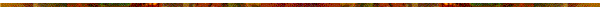• Charles' Law

• V1 / T1 = V2 / T2.

• This equation of V1 / T1 = V2 / T2 will be the basis for solving Charles' Law problems.

•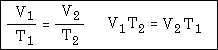• "Notice that the right-hand equation results from cross-multiplying the first one. Some people remember one better than the other, so both are provided."

• Example #1: A gas is collected and found to fill 2.85 L at 25.0°C. What will be its volume at standard temperature?

• Answer: convert 25.0°C to Kelvin and you get 298 K. Standard temperature is 273 K. We plug into our equation like this:

•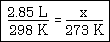• Remember that you have to plug into the equation in a very specific way. The temperatures and volumes come in connected pairs and you must put them in the proper place

• Example #2: 4.40 L of a gas is collected at 50.0°C. What will be its volume upon cooling to 25.0°C?

• First of all, 2.20 L is the wrong answer. Sometimes one is tempted to look at the temperature being cut in half and reason that the volume must also be cut in half. That would be true if the temperature was in Kelvin. However, in this problem the Celsius is cut in half, not the Kelvin.

• Answer: convert 50.0°C to 323 K and 25.0°C to 298 K. Then plug into the equation and solve for x, like this:

•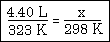• Example #3: 5.00 L of a gas is collected at 100 K and then allowed to expand to 20.0 L. What is the new temperature  in order to maintain the same pressure (as required by Charles' Law)?

• :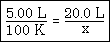• Gay-Lussac's Law

• The equation of P1 / T1 = P2 / T2 is the basis for Gay-Lussac's Law problem solutions

• "Notice the similarities to the Charles' Law graphic. This is because both laws are direct relationships.Make sure to convert any Celsius temperature to Kelvin before using it in your calculation."

• "Example #1: 10.0 L of a gas is found to exert 97.0 kPa at 25.0°C. What would be the required temperature (in Celsius) to change the pressure to standard pressure?

• Answer: change 25.0°C to 298.0 K and remember that standard pressure in kPa is 101.325. Insert values into the equation (the ChemTeam will use the left-hand one in the graphic above) and get:

•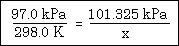• The answer is 311.3 K, but the question asks for Celsius, so you subtract 273 to get the final answer of 38.3°C,

• Example #2: 5.00 L of a gas is collected at 22.0°C and 745.0 mmHg. When the temperature is changed to standard conditions, what is the new pressure? Answer: convert to Kelvin and insert:

•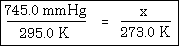Combined Gas Law

•     P1V1 / T1 = P2V2 / T2

• Example #1: This type of combined gas law problem (where everything goes to STP) is VERY common:

• 2.00 L of a gas is collected at 25.0°C and 745.0 mmHg. What is the volume at STP? STP is a common abbreviation for "standard temperature and pressure."

• You have to recognize that five values are given in the problem and the sixth is an x. Also, remember to change the Celsius temperatures to Kelvin. A solution matrix, like this: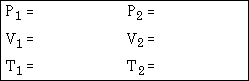•  and fill it in with data from the problem. Here is the right-hand side filled in with the STP values: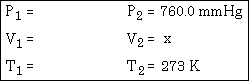•  You can be pretty sure that the term "STP" will appear in the wording of at least one test question in your classroom. Here's the solution matrix completely filled in: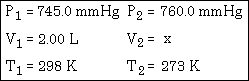•  Insert the values in their proper places in the combined gas law equation: P1V1 / T1 = P2V2 / T2  and solve for xPV = nRT: The Ideal Gas Law

• "Example #1 - A sample of dry gas weighing 2.1025 grams is found to occupy 2.850 L at 22.0°C and 740.0 mmHg. How many moles of the gas are present? Notice that the units for pressure MUST be in atm., so the 740.0 mm Hg must be converted first.  740.0 mm Hg ÷ 760.0 mm Hg/atm = 0.9737 atm

• However, the unrounded-off value should be used in the calculation just below.

• Now, plug into the equation

• (0.9737 atm) (2.850 L) = (n) (0.08206 L atm / mol K) (295.0 K) and solve for n."